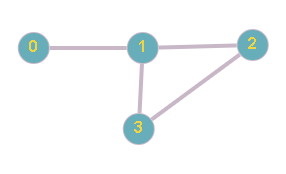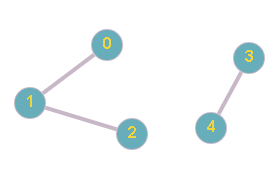Problem title
Difficulty
Avg time to solve

BST Delete
Easy
15 mins
Topological Sorting
Moderate
30 mins
Shortest subarray with sum at least K
Moderate
15 mins
Power Of Power
Easy
15 mins
Minimum days to complete work
Easy
15 mins
Smallest Common Element
Moderate
20 mins
Minimum Time
Moderate
30 mins
Asteroid Collision
Moderate
15 mins
Fact Digit Sum
Easy
15 mins
Check If Two Nodes Are Cousins
Moderate
10 mins3

# Reachable Nodes

Difficulty: EASY
Avg. time to solve
10 min
Success Rate
90%

Problem Statement

#### For example:

``````For given N = 4, M = 4,
````````````In the above example, the number of nodes reachable from nodes 0 , 1, 2 and 3 is 4.
``````
##### Input Format:
``````The first line contains one positive integer ‘T’, denoting the number of test cases, then ‘T’ test cases follows.

The first line of each test case contains two integers ‘N’ and  ‘M’, denoting the number of nodes and the number of edges.

The next ‘M’ lines of each test case contains two space-separated integers ’u’ and ‘v’, denoting the edge between ‘u’ and ‘v’.
``````
##### Output Format:
``````The first line of each test case contains an ‘N’ space separated integer, denoting the number of nodes reachable from node ‘i’, where 0 <= ‘i’ <= ‘N’ - 1.

Output of each test case will be printed on a separate line.
``````
##### Note:
``````You do not need to print anything, it has already been taken care of. Just implement the given function.
``````
##### Constraints:
``````1 <= T <= 5
1 <= N, M <= 10 ^ 3
0 <= u, v <= N - 1

Time Limit: 1 sec.
``````
##### Sample Input 1:
``````2
4 4
0 1
1 2
1 3
2 3
5 3
0 1
1 2
3 4
``````
##### Sample Output 1:
``````4 4 4 4
3 3 3 2 2
``````
##### Explanation of Sample Output1:
``````In the first test case, the graph like this:
````````````As we can observe, The number of reachable nodes from node 0 is 4, i.e 0 to {0, 1, 2, 3}. Also the graph is completely connected. Therefore, all the nodes can be reached from all the other nodes. Hence, the answer is {4, 4, 4, 4}.

In the second test case, the graph looks like this:
````````````As we can observe, there are two disconnected graphs - {0, 1, 2} and {3, 4}. Therefore, The number of reachable nodes from nodes 0,1 and 2 is 3 and from nodes 3 and 4 is 2.
``````
##### Sample Input 2:
``````2
5 4
0 0
0 1
1 3
4 2
2 2
0 0
1 1
``````
##### Sample Output 2:
``````3 3 2 3 2
1 1
``````Console